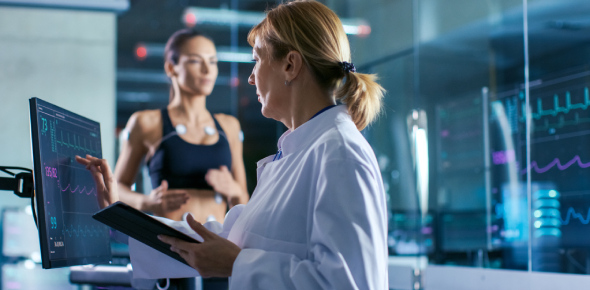# Physical Science MCQ Exam: Quiz!

32 Questions | Total Attempts: 714SettingsPhysical science is all about unraveling the hidden science or factor behind any phenomena. It is a study of matter, energy, and living things that incorporate geology, physics, chemistry, and atmospheric science. This quiz will evaluate your knowledge of physics and chemistry. Read each question carefully and circle the best response. Good Luck!

• 1.
The atoms and molecules that make up a rock.
• A.

Are in constant motion

• B.

Move only when the rock is broken

• C.

Move only when the rock is melted

• D.

Never move because the rock is solid

• 2.
A substance that contains only one type of atom is a(n)
• A.

Compound

• B.

Element

• C.

Heterogeneous mixture

• D.

Homogeneous mixture

• 3.
A sealed metal container holds a gas. What happens when the container is heated?
• A.

The gas exerts more pressure

• B.

The gas decreases in volume

• C.

The mass of the gas increases

• D.

The weight of the gas decreases

• 4.
Which of these is an example of matter?
• A.

The sound of thunder

• B.

Sunlight

• C.

Heat from an oven

• D.

Smoke from a fire

• 5.
How are atoms and elements related?
• A.

Elements can combine to form atoms

• B.

Every element contains only one kind of atom

• C.

All elements contain different types of atoms

• D.

Elements are smaller than the atoms that compose them

• 6.
Suppose you examine an unknown liquid. You determine that it contains a single type of molecule. What is the liquid?
• A.

A pure substance

• B.

A heterogeneous mixture

• C.

A type of matter with a large mass

• D.

A homogeneous mixture

• 7.
When substances combine to make compounds, the proportions of each substance in the compound.
• A.

Are always one to one

• B.

Depend on the amounts of substances

• C.

Change throughout the compound

• D.

Are the same throughout the compound

• 8.
In which of the following states can molecules and atoms move?
• A.

Solid, liquid, or gas

• B.

Liquid or gas only

• C.

Gas only

• D.

Liquid only

• 9.
Mass can be measured in units of?
• A.

Grams

• B.

Newtons

• C.

Cubic centimeters

• D.

Milliliters

• 10.
The amount of matter in a desk is the desk's:
• A.

Volume

• B.

Size

• C.

Mass

• D.

State

• 11.
The process of a solid changing directly to a gas is called?
• A.

Evaporation

• B.

Freezing

• C.

Sublimation

• D.

Condensation

• 12.
Which of these is a chemical change, not a physical change?
• A.

A ball of clay rolled out flat

• B.

A loaf of bread baked in the oven

• C.

A piece of paper torn to shreds

• D.

A bowl of ice cream melted into liquid

• 13.
When a gas changes into a liquid, the process is called?
• A.

Evaporation

• B.

Sublimation

• C.

Chemical change

• D.

Condensation

• 14.
To identify a substance by its density, you must determine its:
• A.

Mass and volume

• B.

Mass and weight

• C.

Shape and volume

• D.

Weight and shape

• 15.
There are two kinds of electric charge. They are:
• A.

Left and right

• B.

Up and down

• C.

Positive and negative

• D.

Physical and chemical

• 16.
Which is an example of a chemical change to matter?
• A.

Ice melting

• B.

Iron rusting

• C.

• D.

Crushing a can

• 17.
When water boils it changes from a:
• A.

Gas to a solid

• B.

Solid to a liquid

• C.

Solid to a gas

• D.

Liquid to a gas

• 18.
The most common element in the universe is:
• A.

Nitrogen

• B.

Hydrogen

• C.

Oxygen

• D.

Aluminum

• 19.
Electrons
• A.

Have a positive charge

• B.

Determine the atomic number of an element

• C.

Have more mass than the other parts of an atom

• D.

Move around outside the nucleus

• 20.
Mg (+2 charge) is a magnesium ion that has:
• A.

Two fewer electrons than a neutral magnesium atom

• B.

Two fewer neutrons than a neutral magnesium atom

• C.

Two more protons than a neutral magnesium atom

• D.

An atomic mass greater than that of a neutral magnesium atom

• 21.
Most elements on the periodic table are:
• A.

Metalloids

• B.

Metals

• C.

Noble gases

• D.

Nonmetals

• 22.
The least active elements are:
• A.

Halogens

• B.

Alkali metals

• C.

Alkaline earth metals

• D.

Noble gases

• 23.
Most nonmetals are:
• A.

Liquid at room temperature

• B.

Poor conductors of heat

• C.

Easy to hammer and shape

• D.

Shiny in appearance

• 24.
The positively charged particles in an atom are:
• A.

Electrons

• B.

Neutrons

• C.

Nuclei

• D.

Protons

• 25.
Nitrogen-14 and Nitrogen-15 are examples of:
• A.

Isotopes

• B.

Ions

• C.

Protons

• D.

Neutrons

Related TopicsBack to top